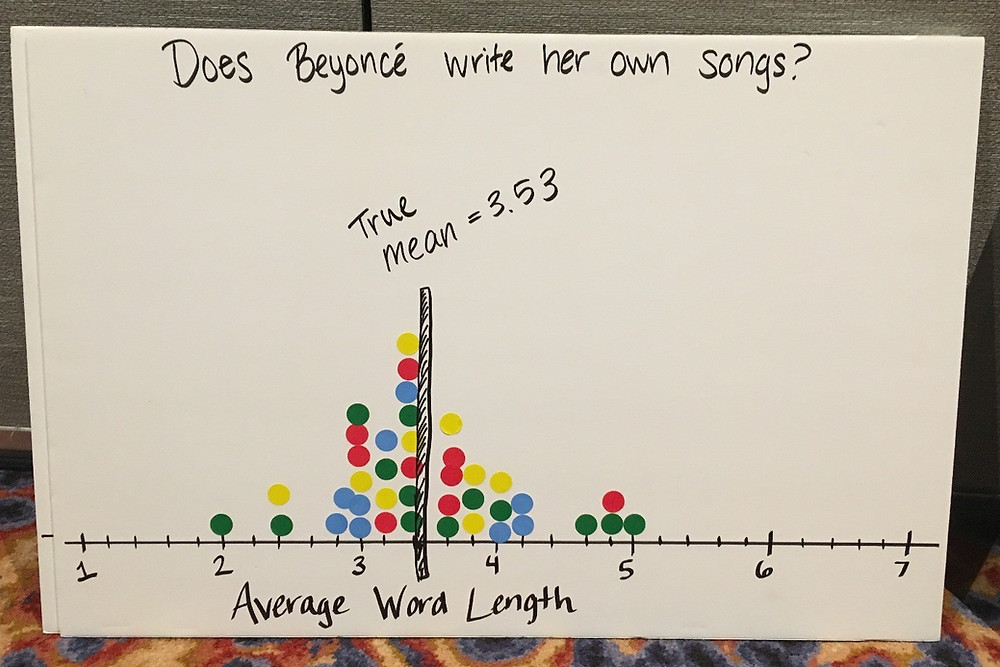Search

# What Does This Dot Represent?

If you have been teaching Introductory Statistics for a bit, you know that inference is the eventual goal at the end of the course. Most important, we want students to be able to use sample data to assess the evidence against a claim, and ultimately make a proper conclusion. We've found that students can be trained to write perfect significance tests without actually grasping what they're writing.

## How Do We Get Students to Understand Inference?

To answer this question, we have to peel back some layers.

(1) To make a proper conclusion, students have to be able to calculate and interpret a P-value.

(2) To calculate a P-value, students have to check conditions and know some formulas for sampling distributions.

(3) To check conditions and know some formulas for sampling distributions, students have to understand the concept of a sampling distribution.

The start of this whole chain reaction is that students need a good fundamental understanding of sampling distributions. No problem. They just need a definition:This definition works well for someone who has experience with sampling distributions (like you!) but is very challenging for introductory students. So how do we give students experience with sampling distributions so that this makes sense?

To calculate a P-value, students are most often using a model (like a Normal distribution, a t-distribution, or a chi-square distribution). Most of the time, this is a continuous model for a distribution of discrete values. So the Normal curve is actually just approximating the distribution of a bunch of possible statistics.

For example, let’s look at the Beyoncé Activity.It certainly seems reasonable to approximate this pile of dots using a Normal curve. But to really understand this picture, students need to understand how each one of these dots is created. This is why we need a simulation. Students need to actually take individual samples and calculate a statistic for each sample. They need to physically walk to the front of the room and put their dot on the dotplot. They are now ready to understand sampling distributions…they just need the teacher to ask the right questions.

## What Does This Dot Represent?

I point to one of the dots (say the green one at 4.6) and ask “What does this dot represent?” After a few students offer their ideas, we eventually refine the response with the perfect answer:

A random sample of 5 words, and a mean calculated from that sample.

I point to a different dot (say the blue one at 2.8) and ask “What does this dot represent?” (emphasis on this). Eventually we arrive at the perfect answer:

A different random sample of 5 words, and a mean calculated from that sample.

And then I point to several points and say

and this is a different random sample and this is a different random sample and this is a different random sample…..and for each sample we calculate a mean.

So each dot represents a random sample of 5 words and a mean calculated for each sample.

## Does This Question Always Work?

Yes! This same set of questions will work for any sampling distribution. The only thing that changes is the “thing” that is calculated from the sample. The “thing” could be a mean, a proportion, a difference of means, a difference of proportions, or a chi-square value. Actually, that “thing” is just a statistic. So we now have a generic answer to the question “What does this dot represent?”

A random sample and a [statistic] calculated from that sample.

Imagine using this line of questioning over and over again well before you get to teaching students sampling distributions. This question could be used in Joy Parkinson’s, Beyonce, Justin Timberlake, and Soda Contest activities, among others. If students had experience with all these simulations and every time they were asked “What does this dot represent?”, don’t you think this will now look different?